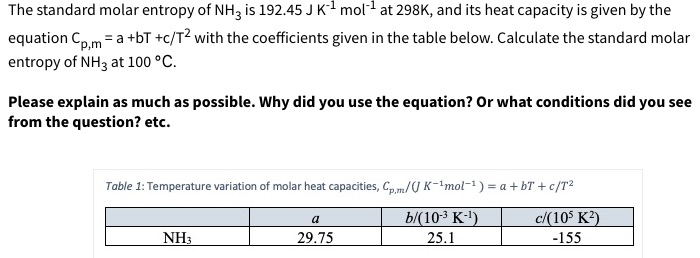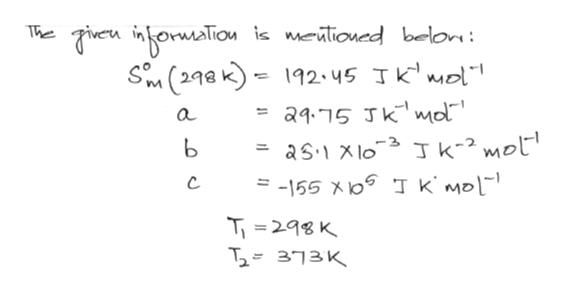# The standard molar entropy of NH3 is 192.45 J K1 mol at 298K, and its heat capacity is given by theequation Coma +bT +c/T2 with the coefficients given in the table below. Calculate the standard molarentropy of NH3 at 100 °Cp,mPlease explain as much as possible. Why did you use the equation? Or what conditions did you seefrom the question? etc.Table 1: Temperature variation of molar heat capacities, Cp,m/U K-1mol-1) a + bT + c/T2b/(10-3 K-)25.1c/(10 K2)aNH329.75-155

Question
91 viewshelp_outlineImage TranscriptioncloseThe standard molar entropy of NH3 is 192.45 J K1 mol at 298K, and its heat capacity is given by the equation Coma +bT +c/T2 with the coefficients given in the table below. Calculate the standard molar entropy of NH3 at 100 °C p,m Please explain as much as possible. Why did you use the equation? Or what conditions did you see from the question? etc. Table 1: Temperature variation of molar heat capacities, Cp,m/U K-1mol-1) a + bT + c/T2 b/(10-3 K-) 25.1 c/(10 K2) a NH3 29.75 -155 fullscreen
check_circle

Step 1help_outlineImage TranscriptioncloseThe Then borwation is matlioued belon: Sm (298 k) 192.45 TKmol = aq.15 JKmo a as xlo3 -155 Xlo k' moL ь Jk2mot T298 K T2313K fullscreen
Step 2
Step 3

### Want to see the full answer?

See Solution

#### Want to see this answer and more?

Solutions are written by subject experts who are available 24/7. Questions are typically answered within 1 hour.*

See Solution
*Response times may vary by subject and question.
Tagged in

### Chemistry